# SCHOOLS OF STATISTICS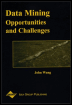Chapter I - A Survey of Bayesian Data Mining Data Mining: Opportunities and Challenges by John Wang (ed) Idea Group Publishing 2003Brought to you by Team-Fly

Statistical inference has a long history, and one should not assume that all scientists and engineers analyzing data have the same expertise and would reach the same type of conclusion using the objectively "right" method in the analysis of a given data set. Probability theory is the basis of statistics, and it links a probability model to an outcome. But this linking can be achieved by a number of different principles. A pure mathematician interested in mathematical probability would only consider abstract spaces equipped with a probability measure. Whatever is obtained by analyzing such mathematical structures has no immediate bearing on how we should interpret a data set collected to give us knowledge about the world. When it comes to inference about real-world phenomena, there are two different and complementary views on probability that have competed for the position of "the" statistical method. With both views, we consider models that tell how data is generated in terms of probability. The models used for analysis reflect our - or the application owner's - understanding of the problem area. In a sense they are hypotheses, and in inference a hypothesis is often more or less equated with a probability model. Inference is concerned with saying something about which probability model generated our data for this reason inference was sometimes called inverse probability (Dale, 1991).

### Bayesian Inference

The first applications of inference used Bayesian analysis, where we can directly talk about the probability that a hypothesis H generated our observed data D. Using probability manipulation and treating both data D and hypotheses H1 and H2 as events we find: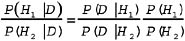This rule says that the odds we assign to the choice between H1 and H2, the prior odds P(H1)/P(H2), are changed to the posterior odds P(H1 D)/P(H 2 D), by multiplication with the Bayes factor P(D H 1)/P(D H 2). In other words, the Bayes factor contains all information provided by the data relevant for choosing between the two hypotheses. The rule assumes that probability is subjective, dependent on information the observer holds, e.g., by having seen the outcome D of an experiment. If we have more than two hypotheses, or a parameterized hypothesis, similar calculations lead to formulas defining a posterior probability distribution that depends on the prior distribution: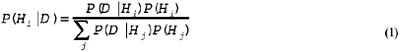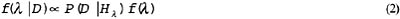where f (λ) is the prior density and f(λD) is the posterior density, and is a sign that indicates that a normalization constant (independent of λ but not of D) has been omitted. For posteriors of parameter values the concept of credible set is important. A q-credible set is a set of parameter values among which the parameter has a high and known probability q of lying, according to the posterior distribution. The Bayes factor estimates the support given by the data to the hypotheses. Inevitably, random variation can give support to the "wrong" hypothesis. A useful rule is the following: if the Bayes factor is k in favor of H1, then the probability of getting this factor or larger from an experiment where H2 was the true hypothesis is less than 1/k. For most specific hypothesis pairs, the bound is much better (Royall, 2000).

### A Small Bayesian Example

We will see how Bayes' method works with a small example, in fact the same example used by Thomas Bayes(1703 1762). Assume we have found a coin among the belongings of a notorious gambling shark. Is this coin fair or unfair? The data we can obtain are a sequence of outcomes in a tossing experiment, represented as a binary string. Let one hypothesis be that the coin is fair, Hf. Then P(D Hf) = 2n, where n=D is the number of tosses made. We must also have another hypothesis that can fit better or worse to an outcome. Bayes used a parameterized model where the parameter is the unknown probability, p, of getting a one in a toss. For this model Hp, we have P(D Hp) = ps(1 p)f for a sequence D with s successes and f failures. The probability of an outcome under Hp is clearly a function of p. If we assume, with Bayes, that the prior distribution of p is uniform in the interval from 0 to 1, we get by Equation (2) a posterior distribution f(pD) = cps(1 p)f, a Beta distribution where the normalization constant is c = (n+1)!/(s!f!). This function has a maximum at the observed frequency s/n. We cannot say that the coin is unfair just because s is not the same as f, since the normal variation makes inequality very much more likely than equality for a large number of tosses even if the coin is fair.

If we want to decide between fairness and unfairness we must introduce a composite hypothesis by specifying a probability distribution for the parameter p in Hp. A conventional choice is again the uniform distribution. Let Hu be the hypothesis of unfairness, expressed as Hp with a uniform distribution on the parameter p. By integration we find P(DHu) =s!f! / (n+1)!. In other words, the number of ones in the experiment is uniformly distributed. Suppose now that we toss the coin twelve times and obtain the sequence 000110000001, three successes and nine failures. The Bayes factor in favor of unfairness is 1.4. This is a too small value to be of interest. Values above 3 are worth mentioning, above 30 significant, and factors above 300 would give strong support to the first hypothesis. In order to get strong support to fairness or unfairness in the example we would need much more than 12 tosses.

### Bayesian Decision Theory and Multiple Hypothesis Comparisons

The posterior gives a numerical measure of belief in the two hypotheses compared. Suppose our task is to decide by choosing one of them. If the Bayes factor is greater than one, H1 is more likely than H2, assuming no prior preference of either. But this does not necessarily mean that H1 is true, since the data can be misleading by natural random fluctuation. The recipe for choosing is to make the choice with smallest expected cost (Berger, 1985). This rule is also applicable when simultaneously making many model comparisons.

When making inference for the parameter value of a parameterized model, equation (2) gives only a distribution over the parameter value. If we want a point estimate l of the parameter value λ, we should also use Bayesian decision theory. We want to minimize the loss incurred by stating the estimate l when the true value is λ, L(l, λ ). But we do not know λ. As with a discrete set of decision alternatives, we minimize the expected loss over the posterior for λ, by integration. If the loss function is the squared error, the optimal estimator is the mean of f(λ D); if the loss is the absolute value of the error, the optimal estimator is the median; with a discrete parameter space, minimizing the probability of an error (no matter how small) gives the Maximum A Posteriori (MAP) estimate. As an example, when tossing a coin gives s heads and f tails, the posterior with a uniform prior is f(ps,f) = cps(1 p)f, the MAP estimate for p is the observed frequency s/(s+f), the mean estimate is the Laplace estimator (s+1)/(s+f+2) and the median is a fairly complicated quantity expressible, when s and f are known, as the solution to an algebraic equation of high degree.

### Test-Based Inference

The irrelevance of long run properties of hypothesis probabilities made one school of statistics reject subjective probability altogether. This school works with what is usually known as objective probability. Data is generated in repeatable experiments with a fixed distribution of the outcome. The device used by a practitioner of objective probability is testing. For a single hypothesis H, a test statistic is designed as a mapping f of the possible outcomes to an ordered space, normally the real numbers. The data probability function P(DH) will now induce a distribution of the test statistic on the real line. We continue by defining a rejection region, an interval with low probability, typically 5% or 1%. Next, the experiment is performed or the data D is obtained, and if the test statistic f(D) falls in the rejection region, the hypothesis H is rejected. For a parameterized hypothesis, rejection depends on the value of the parameter. In objective probability inference, we use the concept of a confidence interval, whose definition is unfortunately rather awkward and is omitted (it is discussed in all elementary statistics texts). Unfortunately, there is no strong reason to accept the null hypothesis just because it could not be rejected, and there is no strong reason to accept the alternative just because the null was rejected. But this is how testing is usually applied. The p-value is the probability of obtaining a test statistic not less extreme than the one obtained, under the null hypothesis, so that a p-value less than 0.01 allows one to reject the null hypothesis on the 1% level.

### A Small Hypothesis Testing Example

Let us analyze coin tossing again. We have the two hypotheses Hf and Hu. Choose Hf, the coin is fair, as the null hypothesis. Choose the number of successes as test statistic. Under the null hypothesis we can easily compute the p-value, the probability of obtaining nine or more failures with a fair coin tossed 12 times, which is .075. This is 7.5%, so the experiment does not allow us to reject fairness at the 5% level. On the other hand, if the testing plan was to toss the coin until three heads have been seen, the p-value should be computed as the probability of seeing nine or more failures before the third success, which is .0325. Since this is 3.25%, we can now reject the fairness hypothesis at 5%. The result of a test depends thus not only on the choice of hypothesis and significance level, but also on the experimental design, i.e., on data we did not see but could have seen.

### Discussion: Objective vs. Subjective Probability

Considering that both types of analysis are used heavily in practical applications by the most competent analysts, it would be somewhat optimistic if one thought that one of these approaches could be shown right and the other wrong. Philosophically, Bayesianism has a strong normative claim in the sense that every method that is not equivalent to Bayesianism can give results that are irrational in some circumstances, for example if one insists that inference should give a numerical measure of belief in hypotheses that can be translated to a fair betting odds (de Finetti, 1974; Savage, 1954). Among stated problems with Bayesian analysis the most important is probably a non-robustness sometimes observed with respect to choice of prior. This has been countered by introduction of families of priors in robust Bayesian analysis (Berger, 1994). Objective probability should not be identified with objective science; good scientific practice means that all assumptions made, like model choice, significance levels, choice of experiment, as well as choice of priors, are openly described and discussed.

Interpretation of observations is fundamental for many engineering applications and is studied under the heading of uncertainty management. Designers have often found statistical methods unsatisfactory for such applications, and invented a considerable battery of alternative methods claimed to be better in some or all applications. This has caused significant problems in applications like tracking in command and control, where different tracking systems with different types of uncertainty management cannot easily be integrated to make optimal use of the available plots and bearings. Among alternative uncertainty management methods are Dempster-Shafer Theory (Shafer, 1976) and many types of non-monotonic reasoning. These methods can be explained as robust Bayesian analysis and Bayesian analysis with infinitesimal probabilities, respectively (Wilson, 1996; Benferhat, Dubois, & Prade, 1997). We have shown that under weak assumptions, uncertainty management where belief is expressed with families of probability distributions that can contain infinitesimal probabilities is the most general method, satisfying compelling criteria on rationality (Arnborg & Sj din, 2001). Most alternative approaches to uncertainty like Fuzzy sets and case-based reasoning can be explained as robust extended Bayesianism with unconventional model families.

 Brought to you by Team-Fly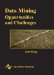Data Mining: Opportunities and Challenges
ISBN: 1591400511
EAN: 2147483647
Year: 2003
Pages: 194
Authors: John Wang